## The Art of the Function

Dec 13

Written by: Charles Flock
12/13/2010 11:01 PMA look at the PV calculation and why it’s easier to use a function even when we can replicate the calculation in T-SQL.
Why are there functions in EXCEL? EXCEL allows you to enter a formula in any cell and almost any function could be entered as a formula. So, why are there functions?
Let’s look at something simple, like the present (PV) value function. The formula for present value is well known, and according the documentation, “Microsoft EXCEL solves for one financial argument in terms of the others. If rate is not 0, then:”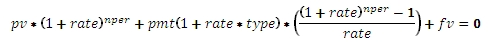“If rate is 0, then”
(pmt * nper) + pv + fv = 0
We can demonstrate this pretty simply: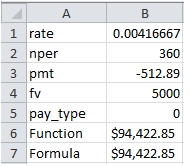In B6 we used the PV function and entered: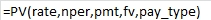In B7 we used the following formula: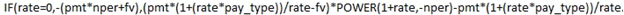So, right away I am voting for simplicity. It seems to me like the function is a lot easier to use than the formula. But we’re not done yet.
It seems like the documentation and the implementation of the function are not actually synchronized. The PV function treats all non-zero values for rate_type as though they are equal to 1. For example, if we change the pay_type to -1, the formula and the function diverge: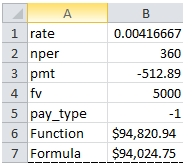Thus we need to make our formula a little more involved: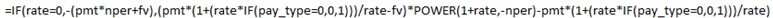Now, the formula matches the function.
Clearly, the function is much simpler to use than the formula. And the formula required that I actually understand more about the present value calculation then I really wanted to, whereas the function just requires that I know the inputs to the function.
The same is true in T-SQL. There is no arithmetic function being used in the PV formula that is not available to us in T-SQL. Let’s translate the formula to T-SQL.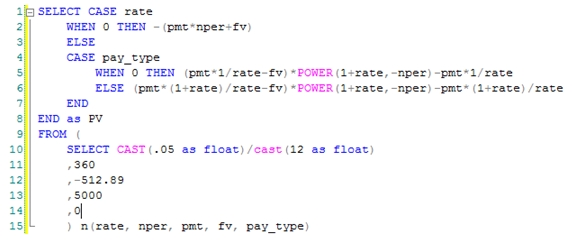This produces the same result as the EXCEL calculation.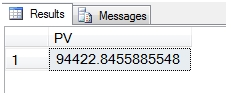Or, you could just enter this: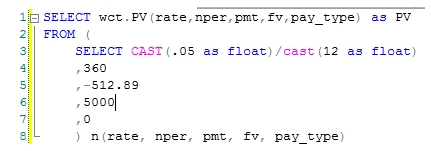Which returns exactly the same result: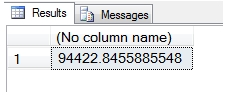If you are using SQL Server 2008 with IntelliSense enabled, SMS will actually prompt you for the input values to the function.
The PV calculation is one of the simpler financial calculations and the math behind it becomes the basis for interest and principal payment calculations and is closely related to the payment calculation and the future value calculation. All of those calculations can be done as formulas, but have been simplified into functions like IPMT, PPMT, PMT, and FV. The math is not particularly hard, but the formulas become longer and that alone makes them more complicated.

I like to keep things simple, and having functions like these makes things a lot simpler. What do you think?

Tags:
Categories:
Location: BlogsThe WestClinTech Blog

## Search Blogs

 KeywordsPhrase

## Blog Archives

Archive
 < September 2023 >
SunMonTueWedThuFriSat
272829303112
3456789
10111213141516
17181920212223
24252627282930
1234567
Monthly
Go

### SupportCopyright 2008-2023 Westclintech LLC         Privacy Policy        Terms of Service